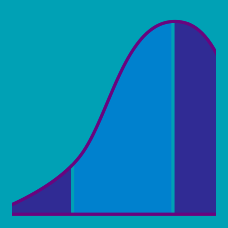Probability

# Continuous Random Variables - Cumulative Distribution Function

If the cumulative distribution function of a continuous random variable $X$ is $F(x)=\begin{cases}0\qquad&(x<0)\\ \frac{1}{50}x^2\qquad&(0\leq x<5)\\ -\frac{1}{50}x^2+\frac{2}{5}x-1\qquad&(5\leq x\leq10)\\ 1\qquad&(x>10) \end{cases}$ what is $P(4\leq X\leq6)?$

If the cumulative distribution function of a continuous random variable $X$ is $F(x)=ax~(0\leq x\leq 9),$ what is $P(1\leq X\leq5)?$

Which of the following represents the graph of the cumulative distribution function of a continuous random variable?

(A)(B)(C)(D)If the cumulative distribution function of a continuous random variable $X$ is $F(x)=a\sqrt{x-3}~(3 which of the following represents the probability density function $f(x)?$

If the probability density function of a continuous random variable $X$ is $f(x)=\frac{a}{x+1}~(0\leq x\leq 4),$ which of the following represents the cumulative distribution function $F(x)?$

×